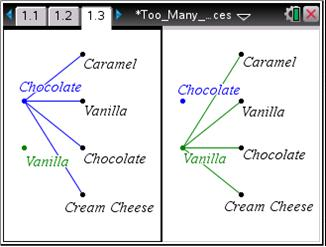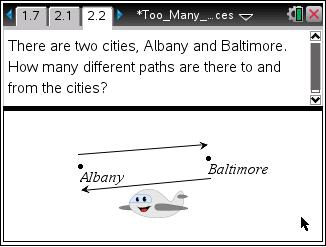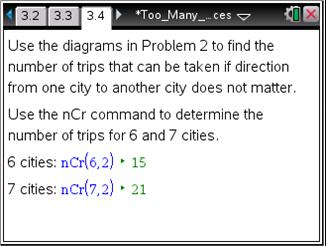### Mathematics lessons for IB® Diploma Programme

Analysis and Approaches | Statistics and Probability

# Too Many Choices

Students investigate the fundamental counting principle, permutations and combinations.

## Key Steps## Step 1

Students will explore the fundamental counting principle and discover its formula. They will use the Segment tool to help them determine the number of different combinations. Each choice is represented as a point.## Step 2

Then, students will use the fundamental counting principle to discover the general formula for permutations. In this investigation students will determine the number of paths that connect 2 cities for a given number of possible cities, where the order of the cities matters.## Step 3

Students will use the general formula for permutations to discover the formula for combinations. They will determine the possible combinations for a committee of 3 people selecting from 6 people. Then students will continue their investigation of the cities by determining the number of trips, where the order of the cities does not matter.